# Problem 56225. Easy Sequences 76: Not so easy as Pisano Pi

Pisano period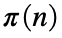, of an integer n, is the period in which the sequence of Fibonacci numbers modulo n repeats. For example it is not hard to show that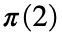,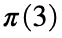and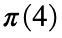are 3, 8 and 6, respectively: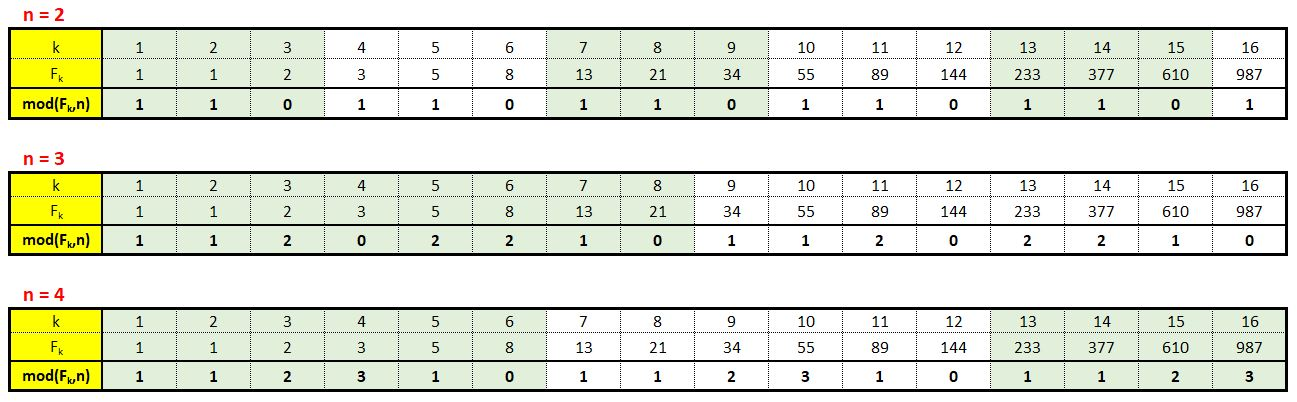This problem is a bit different from the previous Problem 56220. Easy Sequences 75: Easy as Pisano Pi.
In this problem, aside from n, we are given the exponent e and modular base m, and we are asked to calculate:
>> mod(pisanoPi(n^e),m).

### Solution Stats

100.0% Correct | 0.0% Incorrect
Last Solution submitted on Feb 19, 2023

### Community Treasure Hunt

Find the treasures in MATLAB Central and discover how the community can help you!

Start Hunting!